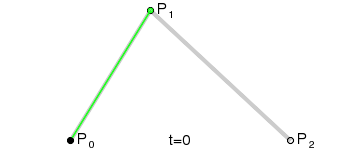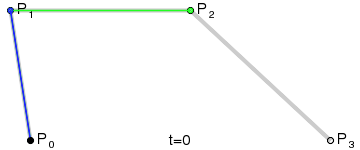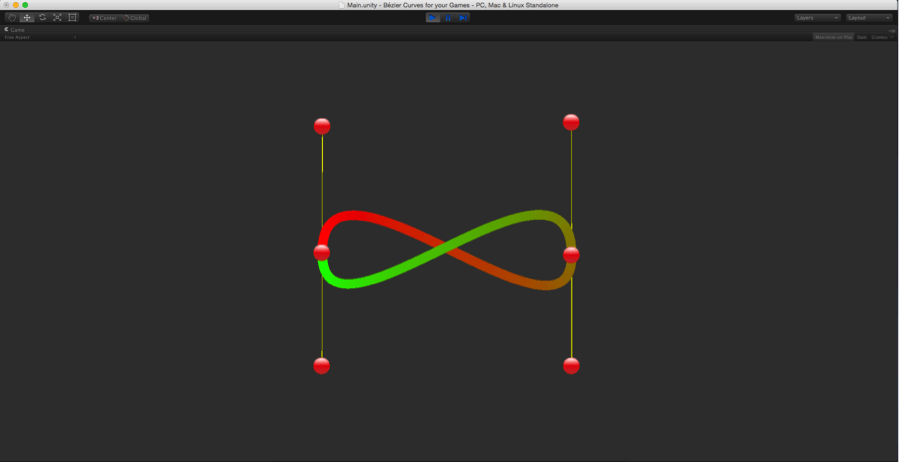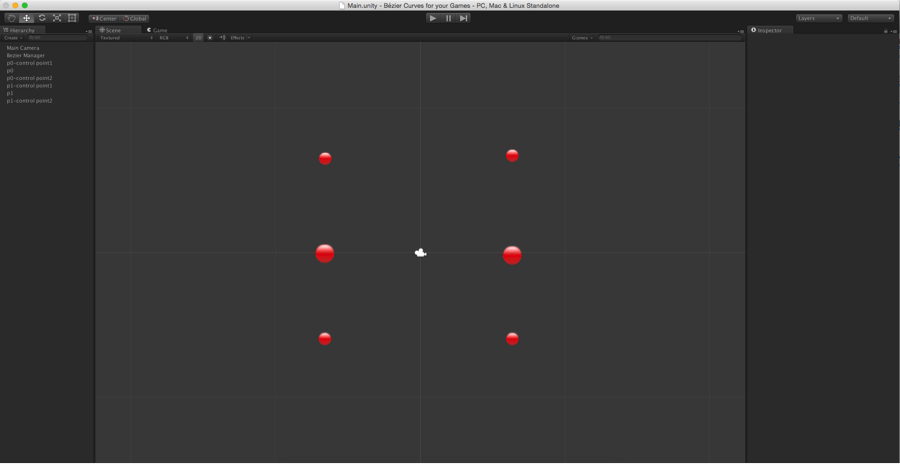Gamasutra is part of the Informa Tech Division of Informa PLC

This site is operated by a business or businesses owned by Informa PLC and all copyright resides with them. Informa PLC's registered office is 5 Howick Place, London SW1P 1WG. Registered in England and Wales. Number 8860726.Latest Jobs
July 16, 2019Latest Blogs
July 16, 2019Press Releases
July 16, 2019
Games PressAboutGama Network
If you enjoy reading this site, you might also want to check out these UBM Tech sites:Blogs

# How to work with Bezier Curve in Games with Unity

by Vivek Tank on 08/06/18 09:48:00 amThe following blog post, unless otherwise noted, was written by a member of Gamasutra’s community.
The thoughts and opinions expressed are those of the writer and not Gamasutra or its parent company.

## Objective

The main objective of this blog post is to give you a basic idea about how to work with Bezier Curve In Games.

## Step 1 Introduction

Bezier curves are the most fundamental curves, used generally in computer graphics and image processing. Bezier curves can be used for creating smooth curved roads, curved paths just like zuma game, curved shaped rivers, etc. in your game.

A Bezier curve is defined by a set of control points P0 through Pn, where n is called its order (n = 1 for linear, 2 for quadratic, etc.). The first and last control points are always the end points of the curve; however, the intermediate control points (if any) generally do not lie on the curve.

• Bezier curve containing two control points i.e. n = 2 is called a Linear Bezier Curve
• Bezier curve containing three control points i.e. n = 3 is called a Quadratic Bezier Curve
• Bezier curve containing four control points i.e. n = 4 is called a Cubic Bezier Curve and so on.

Bezier function, that returns points on bezier curve uses concept of linear interpolation as base. So, Let’s understand what is Linear Interpolation first.

## Step 2 Linear Intrepolation

Linear interpolation between two points means getting interpolated point for different values of t between those two points, where 0 < t < 1, just like Mathf.Lerp.

Formula for interpolated point, P between P0 and P1 can be written as,

• P = P0 + t(P1 – P0)  ,  0 < t < 1

Here, for getting interpolated point we are adding tth fraction of distance between those two points to P0. So,

• For t=0,P = P0.
• For t=1, P = P1.
• For t=0.5, P = Intermediate point between P0 and P1.

## Step 3 Linear Bezier Curves

Linear Bezier curve has two control points. For given two points P0 and P1, a Linear Bezier curve is simply a straight line between those two points. The curve is equivalent to linear interpolation and is given by,

• B(t) = P0 + t(P1 – P0) = (1-t) P0 + tP1 , 0 < t < 1

Animation of how a linear bezier curve is calculated is shown below:## Step 4 Quadratic Bezier Curves

Quadratic bezier curve has three control points. Quadratic Bezier curve is a point-to-point linear interpolation of two Linear Bezier Curves. For given three points P0, P1 and P2, a quadratic bezier curve is a linear interpolation of two points, got from Linear Bezier curve of P0 and P1 and Linear Bezier Curve of P1 and P2. So, Quadratic bezier curve is given by,

• B(t) = (1-t) BP0,P1(t) + t BP1,P2(t), 0 < t < 1
• B(t) = (1-t) [(1-t) P0 + tP1] + t [(1-t) P1 + tP2] , 0 < t < 1

By rearranging the above equation,

• B(t) = (1-t)2P0 + 2(1-t)tP1 + t2P2 , 0 < t < 1

Animation of how quadratic bezier curve is calculated is shown below:## Step 5 Cubic Bezier Curves

Cubic Bezier curve has four control points. Quadratic bezier curve is a point-to-point linear interpolation of two Quadratic Bezier curves. For given four points P0, P1, P2 and P3, a cubic bezier curve is a linear interpolation of two points, got from Quadratic Bezier curve of P0, P1and P2 and Quadratic Bezier Curve of P1, P2 and P3. So, Cubic bezier curve is given by,

• B(t) = (1-t) BP0,P1,P2(t) + t BP1,P2,P3(t), 0 < t < 1
• B(t) = (1-t) [(1-t)2P0 + 2(1-t)tP1 + t2P2] + t [(1-t)2P1 + 2(1-t)tP2 + t2P3]   , 0 < t < 1

By rearranging the above equation,

• B(t) = (1-t)3P0 + 3(1-t)2tP1 + 3(1-t)t2P2 + t3P3 , 0 < t < 1

Animation of how cubic bezier curve is calculated is shown below:So, In general the bezier curve of degree n can be defined as a point-to-point linear interpolation of two points obtained from two corresponding bezier curves of degree n-1.

## Step 6 Demo

In most of applications either quadratic or cubic bezier function is used. However, you can always make use of higher degree bezier function to draw more complicated curves but calculation of higher degree bezier function is more complex and increases processing overhead. So, instead of using higher degree bezier function for drawing more complicated curves, you can use either quadratic or cubic bezier function multiple times. Here, I have created one demo and drawn shape curve, using cubic bezier function two times in a loop as shown below.To create a curve as shown above, create a scene as shown below:Now, attach Bezier.cs script to Bezier Manager.

Bezier.cs:

using UnityEngine;
using System.Collections.Generic;
[RequireComponent(typeof(LineRenderer))]
public class Bezier : MonoBehaviour
{
public Transform[] controlPoints;
public LineRenderer lineRenderer;

private int curveCount = 0;
private int layerOrder = 0;
private int SEGMENT_COUNT = 50;

void Start()
{
if (!lineRenderer)
{
lineRenderer = GetComponent<LineRenderer>();
}
lineRenderer.sortingLayerID = layerOrder;
curveCount = (int)controlPoints.Length / 3;
}

void Update()
{

DrawCurve();

}

void DrawCurve()
{
for (int j = 0; j <curveCount; j++)
{
for (int i = 1; i <= SEGMENT_COUNT; i++)
{
float t = i / (float)SEGMENT_COUNT;
int nodeIndex = j * 3;
Vector3 pixel = CalculateCubicBezierPoint(t, controlPoints [nodeIndex].position, controlPoints [nodeIndex + 1].position, controlPoints [nodeIndex + 2].position, controlPoints [nodeIndex + 3].position);
lineRenderer.SetVertexCount(((j * SEGMENT_COUNT) + i));
lineRenderer.SetPosition((j * SEGMENT_COUNT) + (i - 1), pixel);
}

}
}

Vector3 CalculateCubicBezierPoint(float t, Vector3 p0, Vector3 p1, Vector3 p2, Vector3 p3)
{
float u = 1 - t;
float tt = t * t;
float uu = u * u;
float uuu = uu * u;
float ttt = tt * t;

Vector3 p = uuu * p0;
p += 3 * uu * t * p1;
p += 3 * u * tt * p2;
p += ttt * p3;

return p;
}
}

Here, CalculateCubicBezierPoint function is an implementation of Cubiz Bezier function which I had explained above. DrawCurve function draws two cubic bezier curves.

1. Between P0, P0- control Point1, P1- control Point1 and P1.
2. Between P1, P1- control Point1, P0- control Point2 and P0.

Any control point can handle the curvature of its corresponding curve. You can change the curve at any time by dragging any control point as shown below:I hope you find this blog post is very helpful while working with Bezier Curve in Unity. Let me know in comments if you have any question regarding Unity.